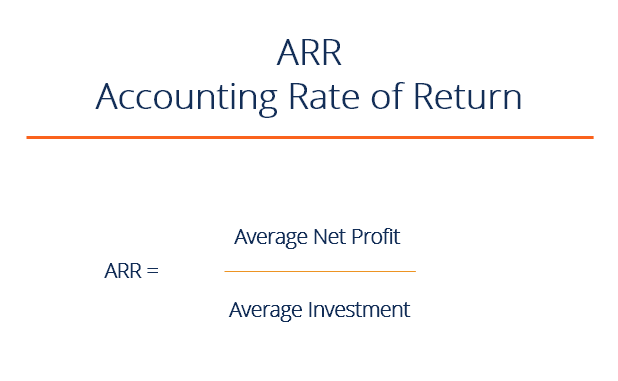# ARR – Accounting Rate of Return

Rate of return from an accounting perspective

## What is ARR?

Accounting Rate of Return (ARR) is the average net income an asset is expected to generate divided by its average capital cost, expressed as an annual percentage. The ARR is a formula used to make capital budgeting decisions. These typically include situations where companies are deciding on whether or not to proceed with a specific investment (a project, an acquisition, etc.) based on the future net earnings expected compared to the capital cost.### ARR formula

The formula for ARR is:

ARR = average annual profit / average investment

Where,

Average investment = (book value at year 1+ book value at end of useful life) / 2

Average annual profit = total profit over investment period/number of years

### Components of Accounting Rate of Return ARR

If the ARR is equal to 5%, this means that the project is expected to earn five cents for every dollar invested per year.

In terms of decision making, if the ARR is equal to or greater than the required rate of return, the project is acceptable because the company will earn at least the required rate of return.

If the ARR is less than the required rate of return, the project should be rejected. Therefore, the higher the ARR, the more profitable the investment.

### ARR – Example 1

XYZ Company is looking to invest in some new machinery to replace its current malfunctioning one. The new machine, which costs \$420,000, would increase annual revenue by \$200,000 and annual expenses by \$50,000. The machine is estimated to have a useful life of 12 years and zero salvage value.

• Calculate the depreciation expense per year: \$420,000 / 12 = \$35,000
• Calculate the average annual profit: \$200,000 – (\$50,000 + \$35,000) = \$115,000
• Use the formula: ARR = \$115,000 / \$420,000 = 27.4%

Therefore, this means that for every dollar invested, the investment will return a profit of about 27 cents.

### ARR – Example 2

XYZ Company is considering investing in a project that requires an initial investment of \$100,000 for some machinery. There will be net inflows of \$20,000 for the first two years, \$10,000 in years three and four, and \$30,000 in year five. Finally, the machine has a salvage value of \$25,000.

Step One: Calculate average annual profit

 \$ Inflows, Years 1 & 2(20,000*2) 40,000 Inflow, Year 3 & 4(10,000*2) 20,000 Inflow, Year 5 30,000 Less: Deprecation(100,000 – 25,000) (75,000) Total Profit of Project 15,000 Average Annual Profit(15,000/5) 3,000

Step Two: Calculate average investment

Average investment = (\$100,000 + \$25,000) / 2  = \$62,500

Step 3: Divide profit into cost

ARR = 3,000/62,500 = 4.8%

### Limitations to Accounting Rate of Return

Although ARR is an effective tool to grasp a general idea of whether to proceed with a project in terms of its profitability, there are several limitations to this approach:

• It ignores the time value of money. It assumes accounting income in future years has the same value as accounting income in the current year. A better metric that considers the present value of all future cash flows is NPV and Internal Rate of Return (IRR).
• It does not consider the increased risk of long-term projects and the increased variability associated with prolonged projects.
• It is only a financial guide for projects. Sometimes projects are proposed and implemented to enhance other important variables such as safety, environmental concerns, or governmental regulations.
• It is not an ideal comparative metric between projects because different projects have different variables such as time and other non-financial factors to consider.

We hope this has been a helpful guide to understanding the Accounting Rate of Return, the formula, and how you can use it in your career.

CFI is a provider of the Financial Modeling and Valuation Certification, a program designed to help anyone become a world-class financial analyst. To keep learning and advancing your career these additional CFI resources will be helpful:

• Internal Rate of Return (IRR)
• Goodwill impairment accounting
• Modified Internal Rate of Return (MIRR)
• Financial modeling guide